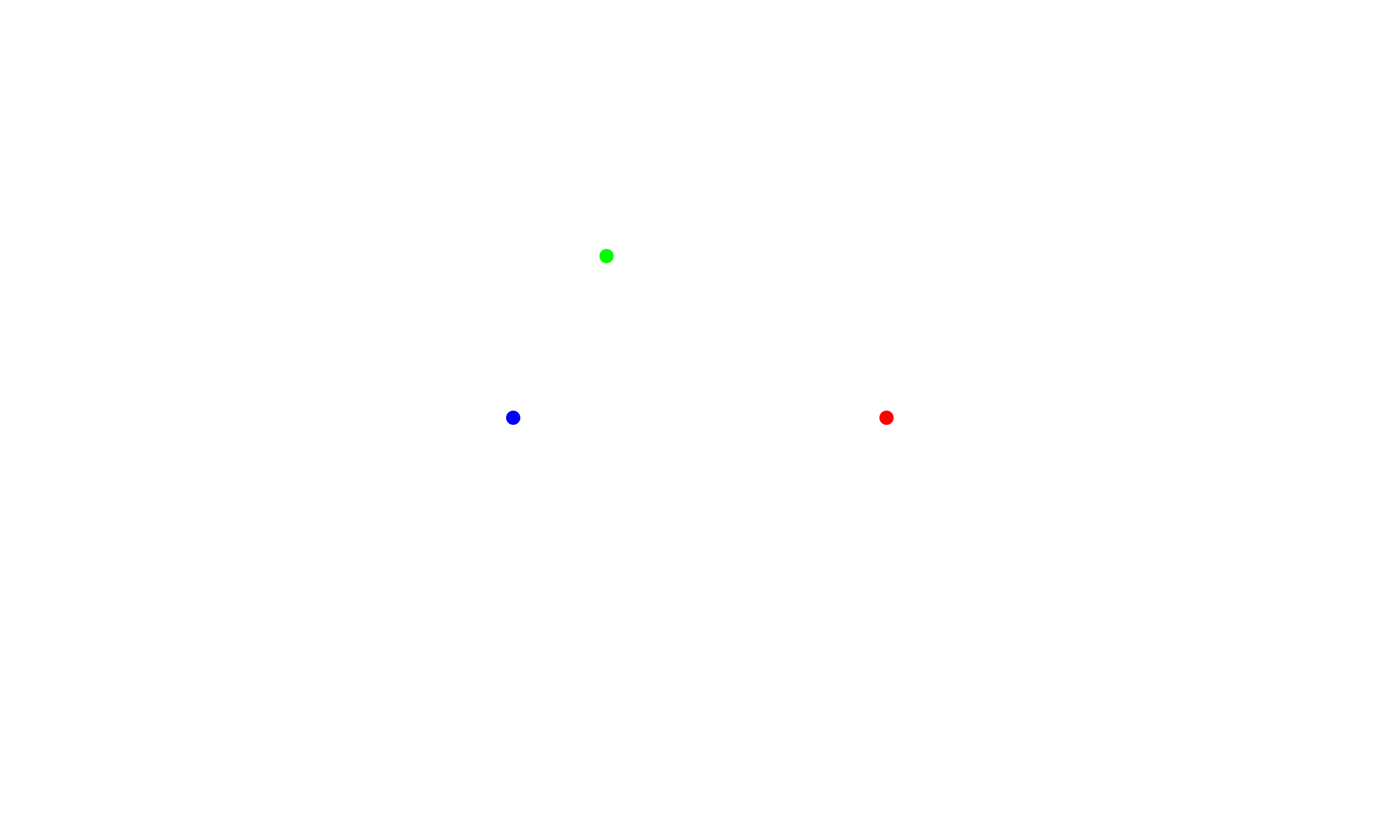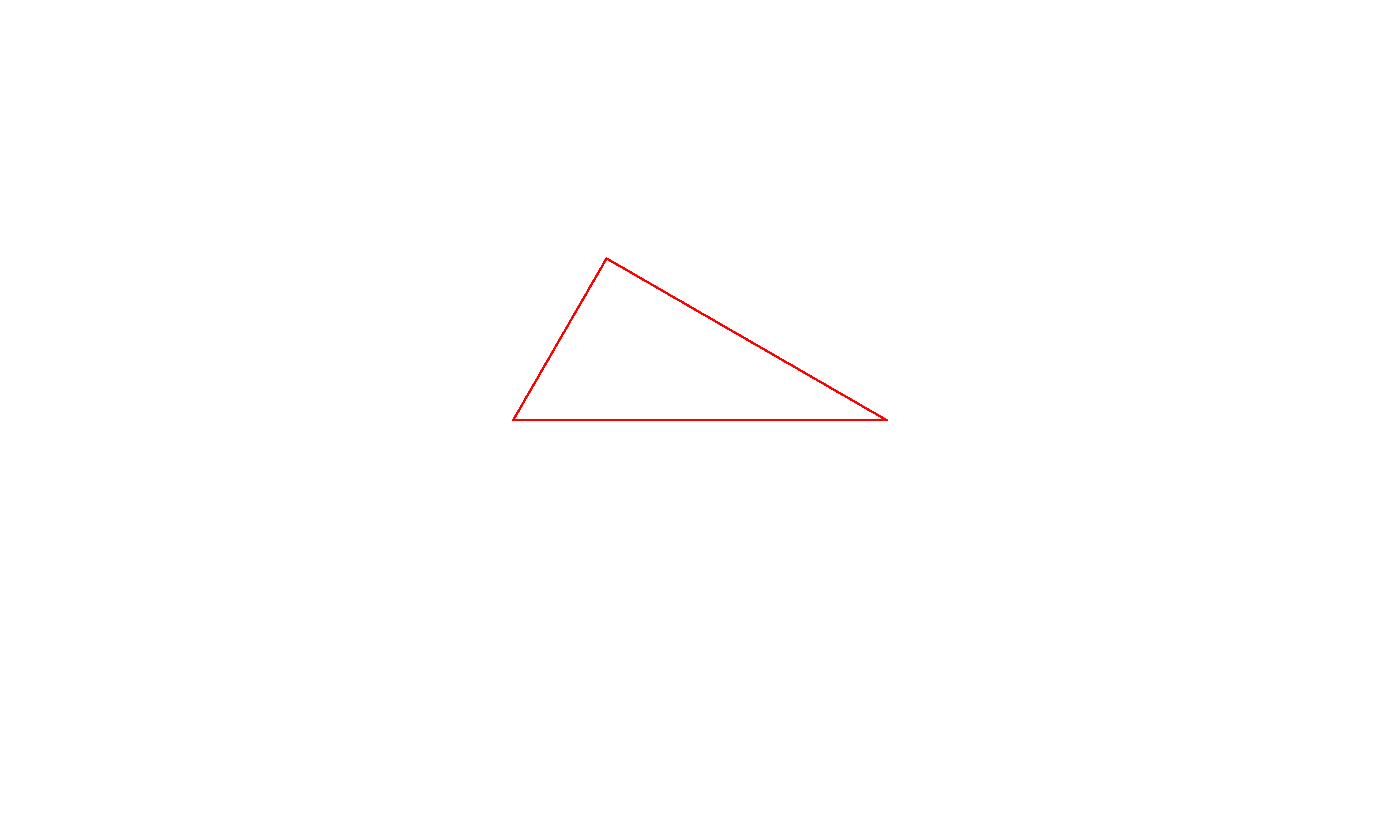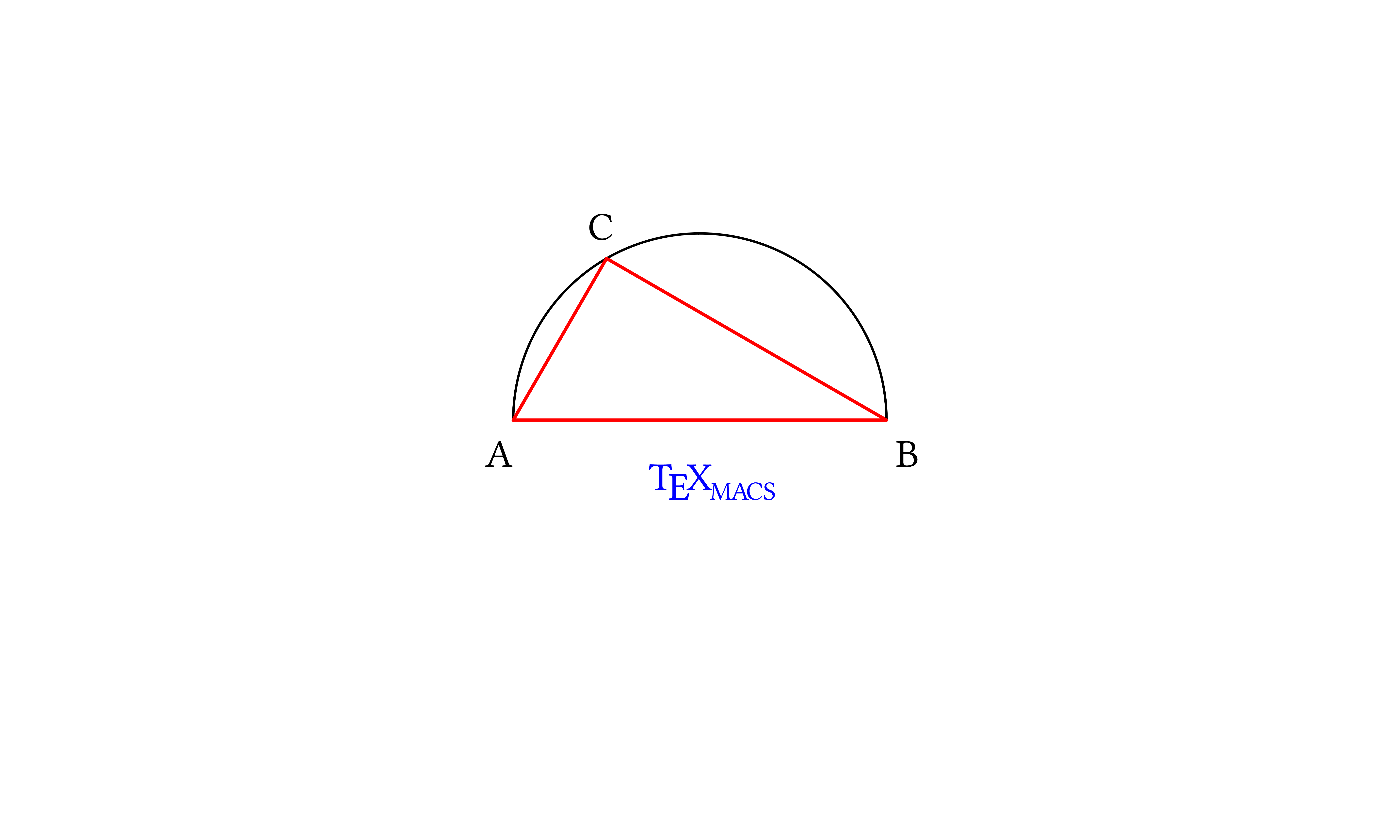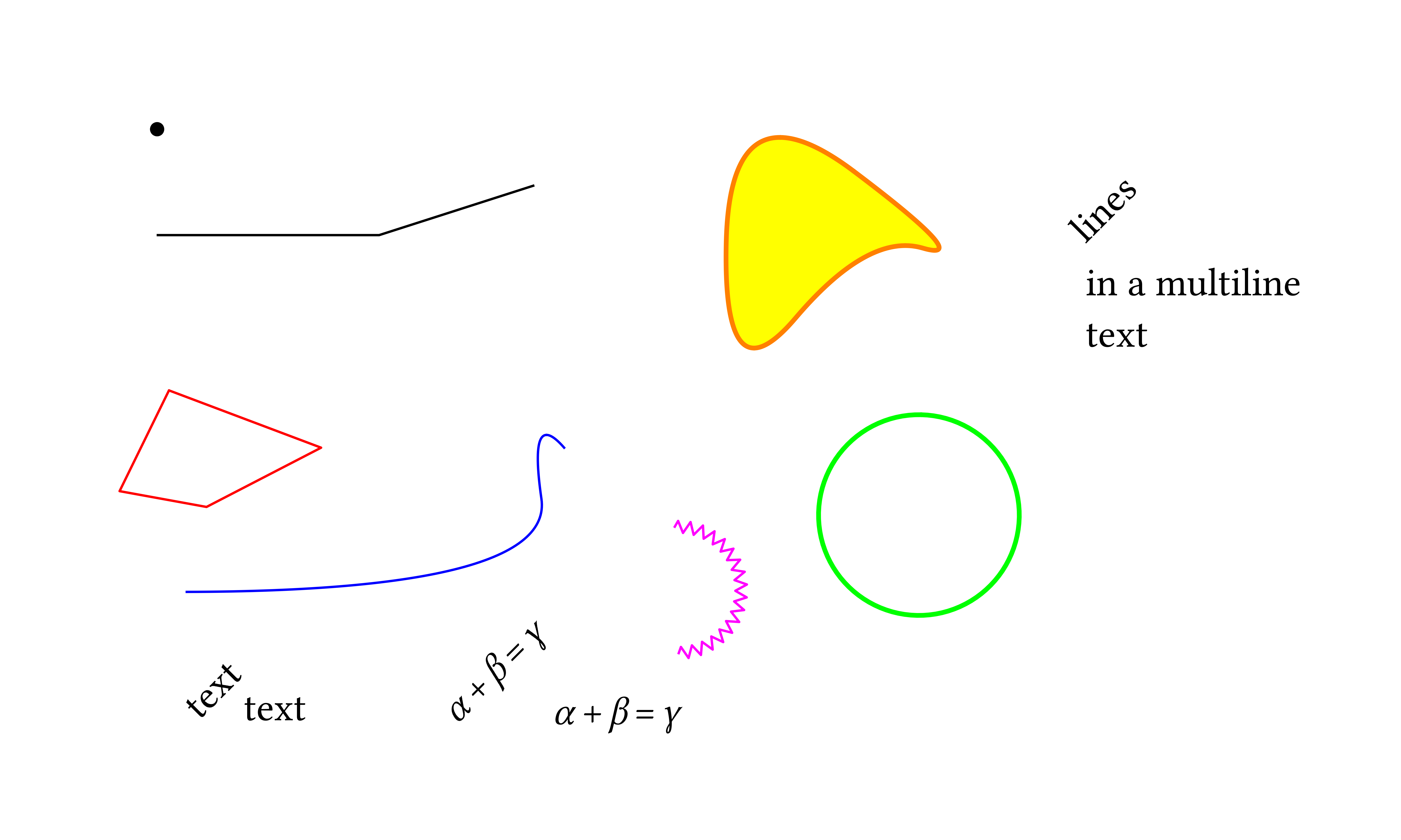[main]Notes on TeXmacs

# Composing TeXmacs graphics with Scheme

TeXmacs provides a set of graphic primitives, which can be accessed in several ways: interactively with the Texmacs editor, directly through the TeXmacs source code (TeXmacs trees) or through Scheme (as Scheme trees). The manual at HelpManualCreating technical pictures describes interactive generation and editing of drawings.

In this note, we shall step through the generation of a simple drawing starting from Scheme code and translating it into a TeXmacs tree which then displays the graphics. We assume that the reader is familiar with simple Scheme syntax. Two possible web resources for learning Scheme are the Wikipedia book Scheme programming and Yet Another Scheme Tutorial by Takafumi Shido.

We want to draw a triangle inscribed inside a semicircle, mark its vertices with letters and decorate the drawing with the text TeXmacs.

The most comfortable way of generating a drawing with Scheme is within a session, so that is the way we'll take in this note. We'll see in other notes how to blend seamlessly graphics in a document and generate it through external source files.

The first step in working with a session is opening it, with InsertSessionScheme. As a side step, it is convenient to select Program bracket matching in the preferences box under EditPreferencesOther; this helps coding in Scheme by highlighting the parenthesis that matches the one next to the cursor.

Now we will insert our commands at the prompt. We place small comments within text fields inserted by choosing Insert text field below in the contextual menu, while longer explanations fit better in breaks between sessions.

 Scheme] `(define pi (acos -1))`

We will work with a circle so we need π!

 Scheme] ```(define (pt x y) ‘(point ,(number->string x) ,(number->string y)))```
 Scheme]

The Scheme function pt we just defined generates a TeXmacs graphics point parametrized by its x and y coordinates.

point is a TeXmacs graphics primitive that represents a point and expects two strings. It is represented in Scheme with a list of three elements, the first element in the list being the symbol point (since point is a Scheme symbol, it fits well within the quasiquote that also defines the list).

Using the pt function we shall now define a few points.

The Scheme interpreter expects one expression per prompt (evaluates only the first one it finds in each prompt), so we enter the expressions we need in separate prompts; the code in external Scheme programs is—of course—more compact.

First of all, coordinates for the end points of the diameter, which is 4 units long while the center of the circle is at the origin:

 Scheme] `(define pA (pt -2 0))`
 Scheme] `(define pB (pt 2 0))`

Then the third point of the triangle, on the circumference, defined with the help of two variables xC and yC:

 Scheme] `(define xC (- (* 2 (cos (/ pi 3)))))`
 Scheme] `(define yC (* 2 (sin (/ pi 3))))`
 Scheme] `(define pC (pt xC yC))`

Finally the points at which we will mark the triangle's vertices with letters:

 Scheme] `(define tA (pt -2.3 -0.5))`
 Scheme] `(define tB (pt 2.1 -0.5))`
 Scheme] `(define tC (pt (- xC 0.2) (+ yC 0.2)))`
 Scheme]

Let us take a look at the points we just defined. To display them as a TeXmacs graphics, we need to insert them in a canvas with the graphics primitive, entered in Scheme as graphics. The Scheme expression that starts with graphics contains, after the symbol graphics, a list of graphical objects; we will then write the canvas with our points as ‘(graphics ,pA ,pB ,pC) with proper quasi- and unquoting (slightly different in the final form of the expression). Since graphics by itself yields a rather large canvas, we size it down enclosing it in a with primitive which specifies the geometry. The (with ... (graphics ...)) construct needs to be quasiquoted as with and graphics are Scheme symbols, so that the pA, pB and pC variables, which represent the points, must be unquoted. Finally, everything has to be wrapped in the stree->tree function to become a TeXmacs tree. The result is a graphical representation of the three points:

 `Scheme] ` ```(stree->tree ‘(with "gr-geometry" (tuple "geometry" "400px" "300px" "center") (graphics ,pA ,pB ,pC)))```We can modify the appearance of the points enclosing each of them in the with (Scheme symbol with) primitive

 `Scheme] ` ```(stree->tree ‘(with "gr-geometry" (tuple "geometry" "400px" "300px" "center") (graphics (with "color" "blue" ,pA) (with "color" "red" ,pB) (with "color" "green" ,pC))))```Scheme]

This example, with constructs boxed inside each other, is typical of Scheme: we compose a list out of other lists. In this case we pass then the list, made of lists, symbols and strings, to the function stree->tree that turns it into a TeXmacs tree.

The next step is composing more complex graphical objects using the points we defined.

We will use the TeXmacs graphical objects arc, line, cline and text-at. Their meaning and Scheme syntax is described in the following table:

 object description Scheme syntax arc an arc of circle, defined by three points (arc point1 point2 point3) line a polyline, defined by two or more points (line point1 point2 [... pointn]) cline a closed polyline, defined by three or more points (cline point1 point2 point3 [... pointn]) text-at a text box, whose position is defined with a single point (text-at string point)

Before composing the full drawing, let us take a look at one of the constructs; as an example we choose cline. We place it as usual inside a with construct to select the color and we wrap the with up in the graphics primitive, which is in turn enclosed in its own with which sets the "gr-geometry" property of the graphics object:

 `Scheme] ` ```(stree->tree ‘(with "gr-geometry" (tuple "geometry" "400px" "300px" "center") (graphics (with "color" "red" (cline ,pA ,pB ,pC)))))```Scheme]

We are ready to compose our drawing; as usual the syntax is

`(graphics object_1 object_2 ... object_n)`

properly enclosed in other constructs (we change the linewidth as well as the color and we set the font shape for the whole graphics to italics), with the appropriate sequence of quasiquoting and unquotings:

 `Scheme] ` ```(stree->tree ‘(with "gr-geometry" (tuple "geometry" "400px" "300px" "center") "font-shape" "italic" (graphics ;; the arc and the line together make the semicircle (with "color" "black" (arc ,pA ,pC ,pB)) (with "color" "black" (line ,pA ,pB)) ;; a closed polyline for the triangle (with "color" "red" "line-width" "1pt" (cline ,pA ,pB ,pC)) ;; add letters using text-at (with "color" "black" (text-at "A" ,tA)) (with "color" "black" (text-at "B" ,tB)) (with "color" "black" (text-at "C" ,tC)) ;; finally decorate with the TeXmacs symbol (with "color" "blue" "font-shape" "upright" (text-at (TeXmacs) ,(pt -0.55 -0.75)))))) ;; and close all of the parentheses!!!```Scheme]

In follow-up tutorials we will see how to embed seamlessly Scheme graphics in a document using the FoldExecutable environment and how to generate them from external files.

As a conclusion of this note, here is a collection of TeXmacs graphical objects, illustrating a few possibilities: